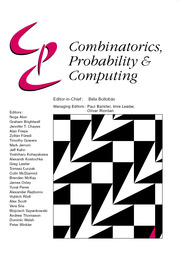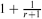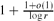Home
Hostname: page-component-cf9d5c678-w9nzq Total loading time: 0.212 Render date: 2021-08-01T08:59:02.606Z Has data issue: true Feature Flags: { "shouldUseShareProductTool": true, "shouldUseHypothesis": true, "isUnsiloEnabled": true, "metricsAbstractViews": false, "figures": true, "newCiteModal": false, "newCitedByModal": true, "newEcommerce": true, "newUsageEvents": true }Combinatorics, Probability and Computing

# Covering Complete r-Graphs with Spanning Complete r-Partite r-Graphs

Published online by Cambridge University Press:  09 February 2011

Corresponding

## Abstract

An r-cut of the complete r-uniform hypergraph Krn is obtained by partitioning its vertex set into r parts and taking all edges that meet every part in exactly one vertex. In other words it is the edge set of a spanning complete r-partite subhypergraph of Krn. An r-cut cover is a collection of r-cuts such that each edge of Krn is in at least one of the cuts. While in the graph case r = 2 any 2-cut cover on average covers each edge at least 2-o(1) times, when r is odd we exhibit an r-cut cover in which each edge is covered exactly once. When r is even no such decomposition can exist, but we can bound the average number of times an edge is cut in an r-cut cover betweenand. The upper bound construction can be reformulated in terms of a natural polyhedral problem or as a probability problem, and we solve the latter asymptotically.

Type
Paper
Information
Combinatorics, Probability and Computing , July 2011 , pp. 519 - 527

## Access options

Get access to the full version of this content by using one of the access options below. (Log in options will check for institutional or personal access. Content may require purchase if you do not have access.)

## References

Alon, N. (1986) Decomposition of the complete r-graph into complete r-partite r-graphs. Graphs Combin. 2 95100.CrossRefGoogle Scholar
Alon, N. and Spencer, J. (2008) The Probabilistic Method, third edition, Wiley Interscience Series in Discrete Mathematics and Optimization.CrossRefGoogle Scholar
Cioabă, S. M. and Kündgen, A. Covering hypergraphs with cuts of minimum total size. Graphs Combin. (to appear).Google Scholar
Cioabă, S. M., Kündgen, A. and Verstraëte, J. (2009) On decompositions of complete hypergraphs. J. Combin. Theory Ser. A 116 12321234.CrossRefGoogle Scholar
Fredman, M. K. and Komlós, J. (1984) On the size of separating systems and families of perfect hash functions. SIAM J. Alg. Discrete Methods 5 6168.CrossRefGoogle Scholar
Füredi, Z. and Kündgen, A. (2001) Covering a graph with cuts of minimum total size. Discrete Math. 237 129148.CrossRefGoogle Scholar
Garey, M., Johnson, D. and So, H. (1976) An application of graph coloring to printed circuit testing. IEEE Trans. Circuits and Systems CAS-23 591599.CrossRefGoogle Scholar
Graham, R. L. and Pollak, H. O. (1971) On the addressing problem for loop switching. Bell System Tech. J. 50 24952519.CrossRefGoogle Scholar
Graham, R. L. and Pollak, H. O. (1972) On embedding graphs in squashed cubes. In Graph Theory and Applications, Vol. 303 of Lecture Notes in Mathematics, Springer, pp. 99110.Google Scholar
Harary, F., Hsu, D. and Miller, Z. (1977) The biparticity of a graph. J. Graph Theory 1 131133.CrossRefGoogle Scholar
Jaeger, F., Khelladi, A. and Mollard, M. (1985) On shortest cocycle covers of graphs. J. Combin. Theory Ser. B 39 153163.CrossRefGoogle Scholar
Jamshy, U. and Tarsi, M. (1989) Cycle covering of binary matroids. J. Combin. Theory Ser. B 46 154161.CrossRefGoogle Scholar
Karlin, S. and Taylor, H. M. (1981) A Second Course in Stochastic Processes, Academic Press.Google Scholar
Klugerman, M., Russell, A. and Sundaram, R. (1998) A note on embedding complete graphs into hypercubes. Discrete Math. 186 289293.CrossRefGoogle Scholar
Kündgen, A. (1998) Covering cliques with spanning bicliques. J. Graph Theory 27 223227.3.0.CO;2-P>CrossRefGoogle Scholar
Kündgen, A. and Spangler, M. (2005) A bound on the total size of a cut cover. Discrete Math. 296 121128.CrossRefGoogle Scholar
Matula, D. W. (1972) k-components, clusters and slicings in graphs. SIAM J. Appl. Math. 22 459480.CrossRefGoogle Scholar
Mehlhorn, K. (1982) On the program size of perfect functions and universal hash functions. In Proc. 23rd Annual IEEE Symposium on Foundations of Computer Science, pp. 170175.Google Scholar
Nilli, A. (1994) Perfect hashing and probability. Combin. Probab. Comput. 3 407409.CrossRefGoogle Scholar
Peck, G. W. (1984) A new proof of a theorem of Graham and Pollak. Discrete Math. 49 327328.CrossRefGoogle Scholar
Radhakrishnan, J. (2001) Entropy and counting. In IIT Kharagpur Golden Jubilee Volume on Computational Mathematics, Modelling and Algorithms (Mishra, J. C., ed.), Narosa, New Delhi.Google Scholar
Tverberg, H. (1982) On the decomposition of K n into complete bipartite graphs. J. Graph Theory 6 493494.CrossRefGoogle Scholar
Vishwanathan, S. (2008) A polynomial space proof of the Graham–Pollak theorem. J. Combin. Theory Ser. A 115 674676.CrossRefGoogle Scholar
2
Cited by

# Send article to Kindle

Note you can select to send to either the @free.kindle.com or @kindle.com variations. ‘@free.kindle.com’ emails are free but can only be sent to your device when it is connected to wi-fi. ‘@kindle.com’ emails can be delivered even when you are not connected to wi-fi, but note that service fees apply.

Find out more about the Kindle Personal Document Service.

Covering Complete r-Graphs with Spanning Complete r-Partite r-Graphs
Available formats
×

# Send article to Dropbox

To send this article to your Dropbox account, please select one or more formats and confirm that you agree to abide by our usage policies. If this is the first time you use this feature, you will be asked to authorise Cambridge Core to connect with your <service> account. Find out more about sending content to Dropbox.

Covering Complete r-Graphs with Spanning Complete r-Partite r-Graphs
Available formats
×

# Send article to Google Drive

To send this article to your Google Drive account, please select one or more formats and confirm that you agree to abide by our usage policies. If this is the first time you use this feature, you will be asked to authorise Cambridge Core to connect with your <service> account. Find out more about sending content to Google Drive.

Covering Complete r-Graphs with Spanning Complete r-Partite r-Graphs
Available formats
×
×